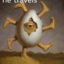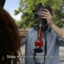MathematicsOpenStudy (anonymous):

Let f(x)=x^2+2x-2 and g(x)=2-3x. Find the following: a.) f(-1) b.) g(-3) c.) f(a+2)OpenStudy (lalaly):

f(-1) =(-1)^2 +2(-1)-2 = 1-2-2 = -3 g(-3) = 2-3(3) = 2-9 = -7 f(a+2) = (a+2)^2 +2(a+2)-2OpenStudy (anonymous):

thank you @lalaly :)OpenStudy (anonymous):

also note that \[f(a+h)=(a+2)^2+2(a+2)-2\] \[=a^2+4a+4+2a+4-2\] \[=a^2+6a+6\]OpenStudy (lalaly):

yesss:DOpenStudy (anonymous):

Okay, thank you @satellite :)OpenStudy (anonymous):

lala, i have a questionOpenStudy (anonymous):

g(-3) = 2-3(3) = 2-9 = -7 isn't it supposed to be 11? because 2-3(-3) = 2+9

Latest QuestionsShelbiLW: A 3.0 m uniform beam of mass 15 kg is pivoted 1.0 m from the end as shown below. A 35 kg child sits 0.
6 hours ago 0 Replies 0 Medalsryleighmullins22: Answer the question below using the following amortization table. Month Principal Paid Interest Paid Balance 21 \$654.
6 hours ago 0 Replies 0 MedalsTonycoolkid21: help
6 hours ago 8 Replies 1 MedalTonycoolkid21: help
6 minutes ago 16 Replies 3 MedalsRx1nyDxyz: Help If the supply of a product increases, then the price decreases*** the price
4 minutes ago 22 Replies 1 Medalshototodorokii: (not from a test/quiz)Nomenclature is governed by certain universal rules. Which
8 hours ago 0 Replies 0 Medalscrispyrat: A guide to sqrt and cbrt
6 hours ago 21 Replies 3 MedalsxXQuintonXx: help please(ss below)
8 hours ago 5 Replies 1 Medalajfknkjfa: Prove that the two circles shown below are similar. (10 points) Circle C is shown with a center at negative 3, 1 and a radius of 4.
8 hours ago 3 Replies 1 Medalrxcklesskaisher: idk wdh dis is
9 hours ago 2 Replies 0 Medals